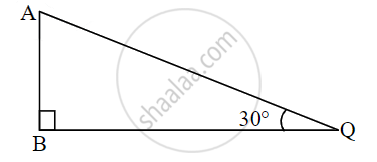# From the given figure, in ∆ABQ, if AQ = 8 cm, then AB =? - Geometry

Sum

From the given figure, in ∆ABQ, if AQ = 8 cm, then AB =?#### Solution

In ∆ABQ,

∠B = 90°, ∠Q = 30°     ...[Given]

∴ ∠A = 60°                  ...[Remaining angle of a triangle]

∴ ∆ABQ is a 30°–60°–90° triangle.

∴ AB = 1/2 AQ         ....[Side opposite to 30°]

∴ AB = 1/2 xx 8

∴ AB = 4 cm

Concept: Right-angled Triangles and Pythagoras Property
Is there an error in this question or solution?
Chapter 2: Pythagoras Theorem - Q.1 (B)

Share